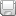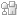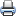# WDM Network DesignSaveDiggDel.icio.usPrint

### Chapter Description

This sample chapter explores some of the design constraints involved in the WDM network design.

## Design of a Point-to-Point Link Based on Q-Factor and OSNR

To design a network, it is imperative to comply the system design with the BER require-ment of the network. If one carefully considers the preceding criteria, it should be evident that calculating BER instantaneously is an intriguing task given that a designer has tools such as a spreadsheet and calculator. Chapter 2 briefly discussed the Q-factor of an optical signal. The Q-factor provides a qualitative description of the receiver performance because it is a function of the signal to noise ratio (optical). The Q-factor suggests the minimum SNR required to obtain a specific BER for a given signal. Figure 4-3 shows the relationship of Q-factor to BER. As we can see, the higher the value of Q-factor, the better the BER.

Figure 4-4 shows the penalty of the Q-factor due to nonlinear effects by increase in input power.Figure 4-3 The Relationship of Q to BERFigure 4-4 Q-Factor Penalty

Mathematically, Equation 4-9 gives the Q-factor of an optical signal.

#### Equation 4-9In Equation 4-9, I1 is the value of the 1-bit current, I0 is the value of the 0-bit current, σ1 is the standard deviation of the 1-bit current, and σ0 is the standard deviation of the 0-bit current. The relationship of Q-factor to BER is shown in Equation 4-10.

#### Equation 4-10A note on error function is provided in Chapter 2.

Why is BER difficult to simulate or calculate? For a given design at a BER (such as 10-12 and a line rate of OC-3, or 155 Mbps), the network would have one error in approximately 10 days. It would take 1000 days to record a steady state BER value. That is why BER calculations are quite difficult. On the other hand, Q-factor analysis is comparatively easy. Q is often measured in dB. The next question is how to dynamically calculate Q. This is done from OSNR.

5. Calculation of Q-Factor from OSNR | Next Section Previous Section